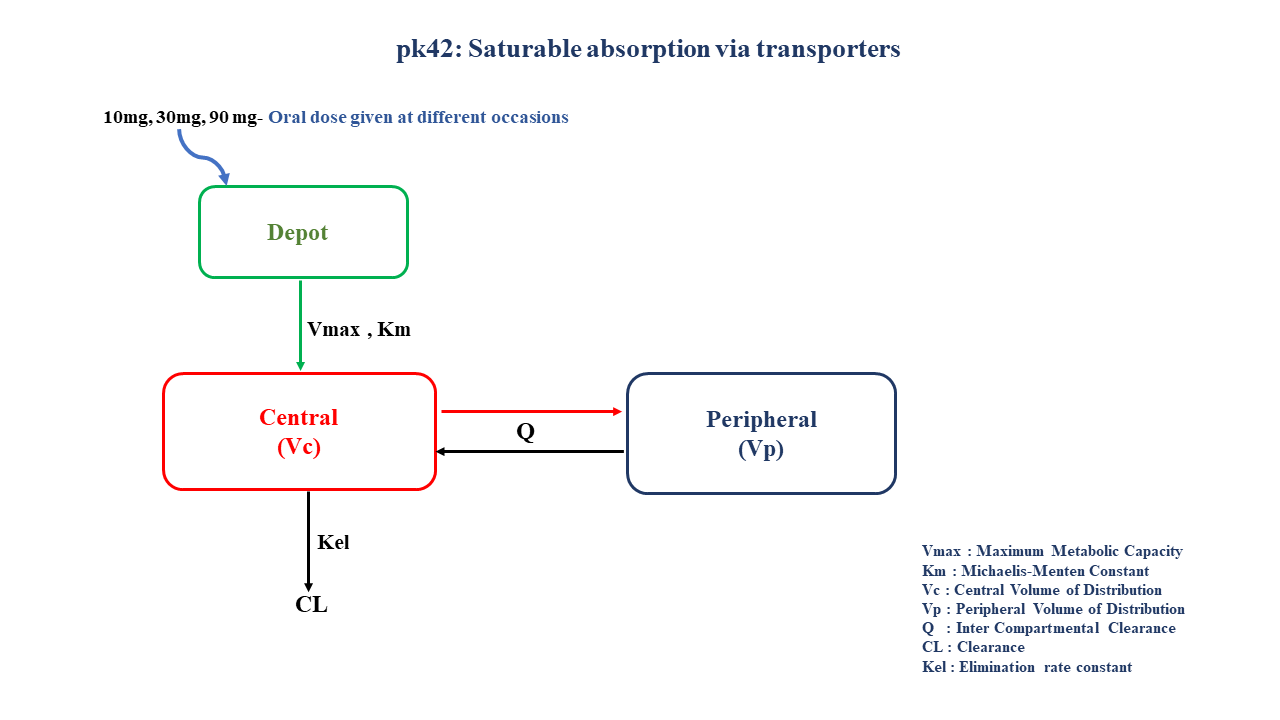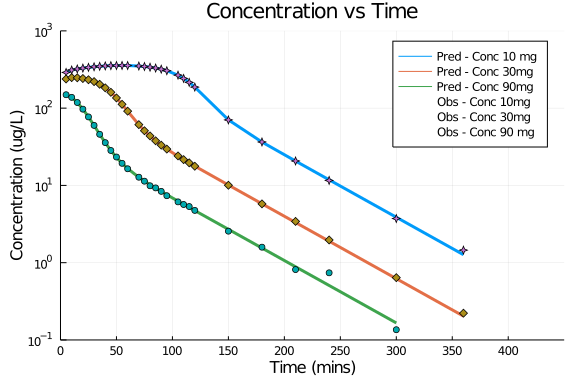# Exercise PK42 - Saturable absorption via transporters

### Background

• Structural model - Multi compartment model with saturable absorption kinetics

• Route of administration - Oral Route

• Dosage Regimen - 10 mg, 30 mg, 90 mg oral dose given at different occasions

• Number of Subjects - 1### Learning Outcomes

This model gives an understanding of non linear absorption due to saturation of drug transporters at higher doses of drug administered orally.

### Objectives

In this tutorial, you will learn to build a multi compartment model for a drug following saturable absorption kinetics and simulate the data.

### Libraries

call the "necessary" libraries to get started.

using Pumas
using Plots
using CSV
using StatsPlots
using Random


### Model

In this multi compartment model,a single subject recieves oral doses of a compound X at three different occasions which follows non linear absorption and linear disposition kinetics.

pk_42           = @model begin
@param begin
tvvmax      ∈ RealDomain(lower=0)
tvkm        ∈ RealDomain(lower=0)
tvvc        ∈ RealDomain(lower=0)
tvvp        ∈ RealDomain(lower=0)
tvq         ∈ RealDomain(lower=0)
tvcl        ∈ RealDomain(lower=0)
Ω           ∈ PDiagDomain(6)
σ_add       ∈ RealDomain(lower=0)
end

@random begin
η           ~ MvNormal(Ω)
end

@pre begin
Vmax        = tvvmax*exp(η)
Km          = tvkm*exp(η)
Vc          = tvvc*exp(η)
Vp          = tvvp*exp(η)
Q           = tvq*exp(η)
CL          = tvcl*exp(η)
end

@vars begin
VMKM       := Vmax/(Km+Depot)
end

@dynamics begin
Depot'      = -VMKM*Depot
Central'    =  VMKM*Depot -CL*(Central/Vc) - (Q/Vc)*Central + (Q/Vp)*Peripheral
Peripheral' = (Q/Vc)*Central - (Q/Vp)*Peripheral
end

@derived begin
cp          = @. Central/Vc
dv          ~ @. Normal(cp,σ_add)
end
end

PumasModel
Parameters: tvvmax, tvkm, tvvc, tvvp, tvq, tvcl, Ω, σ_add
Random effects: η
Covariates:
Dynamical variables: Depot, Central, Peripheral
Derived: cp, dv
Observed: cp, dv


### Parameters

Parameters provided for simulation as below. tv represents the typical value for parameters.

• Vmax - Maximum Metabolic Capacity (ug/min)

• Km - Michaelis-Menten Constant (ug/ml)

• Vc - Volume of Distribution of Central Compartment (L)

• Vp - Volume of Distribution of Peripheral compartment (L)

• Q - Inter Compartmental Clearance (L/min)

• CL - Clearance (L/min)

• Ω - Between Subject Variability

• σ - Residual Error

param = (tvvmax = 982.453,
tvkm   = 9570.63,
tvvc   = 4.66257,
tvvp   = 35,
tvq    = 0.985,
tvcl   = 2.00525,
Ω      = Diagonal([0.0, 0.0, 0.0,0.0,0.0,0.0]),
σ_add  = 0.123)

(tvvmax = 982.453, tvkm = 9570.63, tvvc = 4.66257, tvvp = 35, tvq = 0.985,
tvcl = 2.00525, Ω = [0.0 0.0 … 0.0 0.0; 0.0 0.0 … 0.0 0.0; … ; 0.0 0.0 … 0.
0 0.0; 0.0 0.0 … 0.0 0.0], σ_add = 0.123)


### Dosage Regimen

A single subject received oral dosing of 10,30,90 mg on three different ocassions

ev1      = DosageRegimen(90000, time=0, cmt=1)
sub1     = Subject(id=1, events=ev1, covariates=(Dose="10 mg",))
ev2      = DosageRegimen(30000, time=0, cmt=1)
sub2     = Subject(id=1, events=ev2, covariates=(Dose="30 mg",))
ev3      = DosageRegimen(10000, time=0, cmt=1)
sub3     = Subject(id=1, events=ev3, covariates=(Dose="90 mg",))
pop3_sub = [sub1,sub2,sub3]

Population
Subjects: 3
Covariates: Dose


### Simulation

Simulate the plasma concentration for single subject after an oral dose given at three different occasions.

Random.seed!(123)
sim_pop3_sub = simobs(pk_42, pop3_sub, param, obstimes=0.1:0.1:360)
df1          = DataFrame(sim_pop3_sub)


### Dataframe & Plot

Use the dataframe for plotting

df1_10 = filter(x -> x.Dose == "10 mg", df1)
filter!(x -> x.time in [0,5,10,15,20,25,30,35,40,45,50,55,60,70,75,80,85,90,95,105,110,115,120,150,180,210,240,300,360], df1_10)
df1_30 = filter(x -> x.Dose == "30 mg", df1)
filter!(x -> x.time in [0,5,10,15,20,25,30,35,40,45,50,55,60,70,75,80,85,90,95,105,110,115,120,150,180,210,240,300,360], df1_30)
df1_90 = filter(x -> x.Dose == "90 mg", df1)
filter!(x -> x.time in [0,5,10,15,20,25,30,35,40,45,50,55,60,70,75,80,85,90,95,105,110,115,120,150,180,210,240,300], df1_90)

@df df1_10 plot(:time, :cp, yaxis=:log,
title="Concentration vs Time", label="Pred - Conc 10 mg",
xlabel="Time (mins)", ylabel="Concentration (ug/L)", linewidth=3,
xticks = [0,50,100,150,200,250,300,350,400], yticks = [0.1,1,10,100,1000], xlims=(0,450), ylims=(0.1,1000))
@df df1_30 plot!(:time, :cp, label="Pred - Conc 30mg", linewidth=3)
@df df1_90 plot!(:time, :cp, label="Pred - Conc 90mg", linewidth=3)
@df df1_10 scatter!(:time, :dv, label="Obs - Conc 10mg", markershape=[:star4])
@df df1_30 scatter!(:time, :dv, label="Obs - Conc 30mg", markershape=:diamond)
@df df1_90 scatter!(:time, :dv, label="Obs - Conc 90 mg")